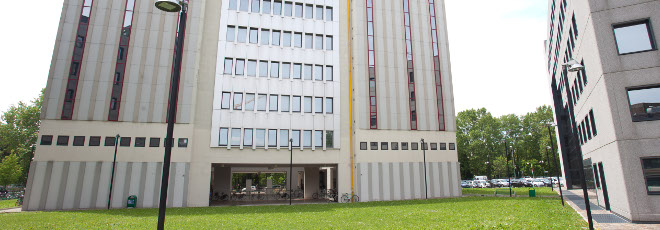## “An energy functional for $L^1$-optimal transport”

Giovedì 30 Aprile 2020, ore 11:30 - Zoom - Federico Piazzon (Università di Padova)

Abstract

The optimal transport problem looks for the minimum cost strategy for moving a given mass distribution to a given target configuration. The case of $L^1$ cost has a PDE reformulation, due to Evans and Gangbo, in which one first searches for the transport density $\mu^*$ and a Kantorovich potential $u^*$ that solve a constrained elliptic PDE. Then the transport plan is constructed by moving the mass along $\nabla u^*$ with intensity $\mu^*$.

We introduce a convex energy functional $\mathcal E$ whose unique minimizer is precisely the transport density, and for this reason we term it transport energy.

The functional $\mathcal E$ is designed upon the structure of the Evans-Gangbo PDE and is inspired by the Bouchitté-Buttazzo-Seppecher shape optimization problem. We construct a metric gradient flow for $\mathcal E$ and characterize $\mu^*$ as its unique global attractor.

We study the variational approximation ($\Gamma$-convergence) of $\mathcal E$ by a double sequence of strictly convex functionals $\mathcal E_{\lambda,\delta}$ and the properties of the resulting $L^2$-gradient flows. In particular we prove existence and uniqueness of the solutions of the resulting fully non-linear evolution systems of PDEs and the convergence of trajectories to the minimizers.

We then develop a numerical approximation scheme based on finite elements for the discretization of the $\mu$ and $u$ functional spaces and relying on the minimizing movements strategy for the minimization of the discretized transport energy. We prove the convergence of the scheme and find a Ladyzhenskaya-Babuska-Brezzi inf-sup-like condition that ensures stability and guarantees quadratic convergence of the overall Newton-based algorithm.

Joint work with Enrico Facca and Mario Putti.

Seminari di equazioni differenziali e applicazioni